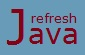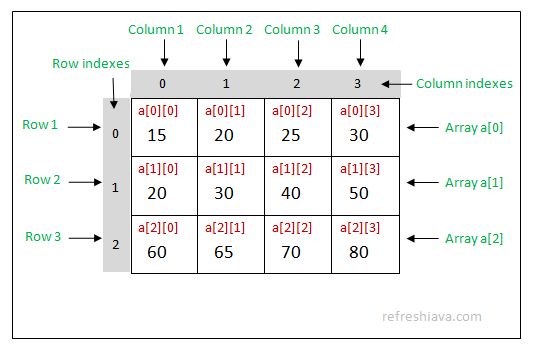# Java Multidimensional Arrays

In java it is possible to define an array whose elements are itself an array. Such arrays are called multidimensional array. A multidimensional array is an array of arrays which simply means the elements of such arrays will itself be an array.

Multidimensional array could be of different types like two dimensional(2D), three dimensional(3D), four dimensional(4D) and so on. In this tutorial we will cover only two and three dimensional array. After the tutorial you will have enough idea to try other dimensional array as well if you want.

## Java Two Dimensional or 2D Arrays

This is the simplest form of multidimensional array. A 2D array is an array of one dimensional arrays which means each elements of two dimensional array will be a single dimensional array.

A 2D array has two dimension which is also called as row and column of 2D array. This type of array has two indexes row index and column index. You can access or assign value in 2D array using the combination of row and column indexes. Row and column indexes starts from `0`. 2D array is similar to matrixes in mathematics where we have rows and columns.

### How to declare 2D arrays

The syntax of declaring 2D array is :

``` `DataType`[][] arrayName;

Example:

`int`[][] intArray; ` // declares a 2D array of integer with name as intArray`
`double`[][] doubleArray; ` // declares a 2D array of double with name as doubleArray`
`char`[][] charArray; ` // declares a 2D array of char with name as charArray````

The double brackets [][] indicates that it's a 2D array. You can use this bracket after the data type or variable name as given in the previous tutorial. The data type can be primitive or non primitive while the name of of array is given as per the programmer's choice.

### How to initialize 2D arrays

``` `// First approach `
`int`[][] matrix =  `new int`;

matrix = 10;
matrix = 20;
matrix = 30;
matrix = 15;
matrix = 80;
matrix = 50;

`// 2nd approach `
`int`[][] a = {{15,20,25,30},{20,30,40,50},{60,65,70,80}};	 ```

In first approach the value given in first bracket `[]` represents number of rows while value given in second bracket `[]` represents number of columns. So in code `new int`, number of rows is 2 and number of column is 3.

In second approach each arrays inside the declaration represents a row while elements inside each array represents number of columns. So the number of rows in 2nd approach is 3 and number of columns is 4.

Each arrays(rows) in second approach can have different number of values(columns). For example 1st array may contain 4 elements, 2nd array may contains 2 elements and 3rd array may contains 3 elements. Such arrays are called jagged arrays. Following example represents a jagged array.

` `int`[][] a = {{15,20,25,30},{20,30},{60,65,70}};	 `

Since 2D array is an array of 1D arrays, so you can think of the arrays given in `{{15,20,25,30},{20,30,40,50},{60,65,70,80}};` like below

` ` {a,a,a};`	 `

Here `a, a, a` are arrays itself which points the corresponding arrays. Similarly in declaration ``` int[][] matrix = new int;``` `matrix` and `matrix` will be an array. The image below displays how 2D array can be represented in rows and columns.To access values in 2D arrays you need to use it's row and column indexes like `a, a, a` and so on. In above example `a` will return `15`, `a` will return `20` and `a` will return `25`.

### What is the length of 2D array ?

The length of 2D array is the total number of arrays declared inside it. In other way the total number of rows inside a 2D array is the length of 2D array. For example in declaration `int[][] a = {{15,20,25,30},{20,30,40,50},{60,65,70,80}}; `length is 3 while in declaration `new int` length is 2.

### What is the total number of elements that can be stored in 2D array ?

To find the total number of elements that can be stored in 2D arrays, you can multiply number of rows and columns. For example in declaration `int[][] matrix = new int`, total number of elements that can be stored is 4x5 = 20.

### 2D Array program in Java

```` class` TwoDArray
{
`public static void` main(String [] args)
{
`int`[][] matrix = `new int`;
matrix = 10;
matrix = 20;
matrix = 30;
matrix = 40;

System.out.println(`"Element at index 00 = "`+matrix);
System.out.println(`"Element at index 01 = "`+matrix);
System.out.println(`"Element at index 10 = "`+matrix);
System.out.println(`"Element at index 11 = "`+matrix);

`int`[][] a = {{15,20,25},{20,30,40},{50,60,70}};

System.out.println(`"\nElement at index 00 = "`+a);
System.out.println(`"Element at index 01 = "`+a);
`// Accessing array elements using for loop`;
System.out.println(`"\nAccessing array element using for loop ........"`);
`for`(`int` i=0; i < a.length; i++)
{
`for`(`int` j=0; j < a[i].length; j++)
System.out.println(`"Element at index "`+i+""+j+`" = "`+a[i][j]);
}
}
}```

Output:

Element at index 00 = 10
Element at index 01 = 20
Element at index 10 = 30
Element at index 11 = 40

Element at index 00 = 15
Element at index 01 = 20

Accessing array element using for loop ........
Element at index 00 = 15
Element at index 01 = 20
Element at index 02 = 25
Element at index 10 = 20
Element at index 11 = 30
Element at index 12 = 40
Element at index 20 = 50
Element at index 21 = 60
Element at index 22 = 70

## Java Three Dimensional or 3D Arrays

A three dimensional array is an array of 2D arrays, which means each elements in 3D array will be a 2D array. Each elements in this array is also accessed by it's indexes.

### How to declare 3D arrays

The syntax of declaring 3D array is :

``` `DataType`[][][] arrayName;

Examples:

`int`[][][] intArray; ` // declares a 3D array of integer with name as intArray`
`double`[][][] doubleArray; ` // declares a 3D array of double with name as doubleArray````

Every declaration in this array is same as 2D array except one more [] bracket. The triple [][][] indicates that it's a 3D array.

### How to initialize 3D arrays

``` `// First approach `
`int`[][][] matrix =  `new int`;

matrix = 10;
matrix = 20;
matrix = 30;
matrix = 40;
matrix = 80;
matrix = 90;
matrix = 15;
matrix = 25;

`// 2nd approach `
`int`[][][] a = {{{15,20},{30,40}},{{25,50},{60,80}}};	 ```

### What is the length of 3D array ?

The length of 3D array is the total number of 2D arrays declared inside it. For example in declaration ` int[][][] a = {{{15,20},{30,40}},{{25,50},{60,80}}}; `length is 2 while in declaration `new int` length will be 2.

### What is the total number of elements that can be stored in 3D array ?

To find the total number of elements that can be stored in 3D arrays, just multiply the values given in [] brackets. For example in declaration `int[][][] matrix = new int`; total number of elements that can be stored is 4x5x2 = 40.

### 3D Array program in Java

```` class` ThreeDArray
{
`public static void` main(String [] args)
{
`int`[][][] a = {{{15,20},{30,40}},{{25,50},{60,80}}};

System.out.println(`"Element at index 000 = "`+a);
System.out.println(`"Element at index 001 = "`+a);
`// Accessing 3D array elements using for loop`;
System.out.println(`"\nAccessing 3D array elements using for loop ........"`);
`for`(`int` i=0; i < a.length; i++)
{
`for`(`int` j=0; j < a[i].length; j++)
{
`for`(`int` k=0; k < a[i][j].length; k++)
System.out.println(`"Element at index "`+i+`""`+j+`""`+k+`" = "`+a[i][j][k]);
}
}
}
}```

Output:

Element at index 000 = 15
Element at index 001 = 20

Accessing array element using for loop ........
Element at index 000 = 15
Element at index 001 = 20
Element at index 010 = 30
Element at index 011 = 40
Element at index 100 = 25
Element at index 101 = 50
Element at index 110 = 60
Element at index 111 = 80